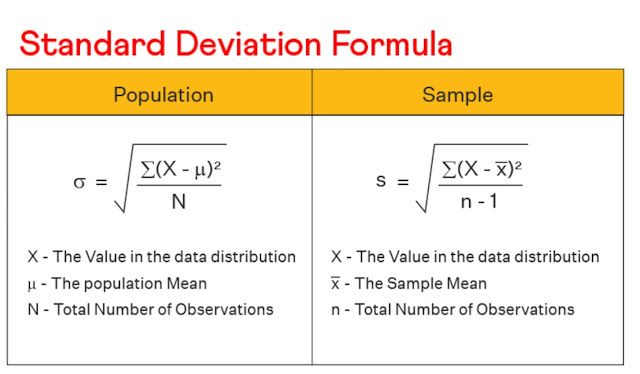# Measures Of Dispersion Class 11 Mathematics Solutions | Exercise - 13.1## Chapter - 13  Measures Of Dispersion

The state of getting dispersed or spread is known as dispersion and the measure of dispersion is a statistic that describes how spread out the values in a data set are. To simplify the term, measure of dispersion shows how squeezed or scattered the variable is. It is typically used to complement a measure of central tendency, such as the mean or median, in describing a data set.

In this chapter, we will learn different methods of finding arithmetic mean, median, standard deviation and coefficient of variation. In addition that, we will get to know about the meaning of dispersion, absolute measure, relative measures, variance etc. Before we move towards the solution section, I would like to give you a brief overview of the main topics covered in this chapter.

### Introduction

The measures which condense the huge mass of data into a single value representing all of theth and around which most of the data tend to concentrate are known as averages. Measure of such single value is known as measure of central tendency.

Of the various measures of central tendency, we deal here briefly only those which are frequently used in the subsequent chapters. The measures of dispersion helps to interpret the variability of data. It means we will be able to know how much homogenous or heterogeneous the data is. There are two types of dispersion which are Absolute Measure and Relative Measure of Dispersion.

### Absolute and Relative Measures

Those measures of dispersion whose units are same as the units of the given series are known as the absolute measure of dispersion. These types of dispersions can be used only comparing the variability of the series (or distribution) having the same units. Comparison of two distributions with different units can not be made with absolute measures.

On the otherhand, the relative measures of dispersions are obtained as the ratio of absolute measure of dispersion to suitable average and are thus a pure number independent of units. Hence t distributions with different units can be compared with the help of relative measures of dispersion.

### Methods of measuring dispersion

The following are the methods of measuring dispersion.
i. Range
ii. Semi interquartile range or Quartile deviation
iii. Mean deviation or Average deviation
iv. Standard deviation

### Requisites of a good measure of dispersion

The good measure of dispersion must have the following characteristics:
1. The measure should be rigidly defined.
2. The measure should be simple to understand and easy to calculate.
3. All items must be included in the measure.
4. The measure must be suitable for further mathematical treatment.
5. Fluctuation of sampling in the measure should be least.
6. Extreme values should not unduly affect the measure.### Exercise - 13.1

In this PDF, you'll only find the solution of class 11 measures of dispersion chapter. It contains all the solutions of exercise- 13.1. If you want the solutions of other exercises then you'll find them above this PDF. Just click on the button and you'll reach your destination.

NoteScroll the PDF to view all Solution

You are not allowed to post this PDF in any website or social platform without permission.

## Is Class 11 Mathematics Guide Helpful For Student ?

I have published this Notes for helping students who can't solve difficult maths problems. Student should not fully depend on this note for completing all the exercises. If you totally depend on this note and simply copy as it is then it may affect your study.

Student should also use their own will power and try to solve problems themselves. You can use this mathematics guide PDF as a reference. You should check all the answers before copying because all the answers may not be correct. There may be some minor mistakes in the note, please consider those mistakes.

## How to secure good marks in Mathematics ?

As, you may know I'm also a student. Being a student is not so easy. You have to study different subjects simultaneously. From my point of view most of the student are weak in mathematics. You can take me as an example, I am also weak in mathematics. I also face problems while solving mathematics questions.

If you want to secure good marks in mathematics then you should practise them everyday. You should once revise all the exercise which are already taught in class. When you are solving maths problems, start from easy questions that you know already. If you do so then you won't get bored.

Maths is not only about practising, especially in grade 11 you to have the basic concept of the problem. When you get the main concept of the problem then you can easily any problems in which similar concept are applied.

When your teacher tries to make the concept clear by giving examples then all students tries to remember the same example but you should never do that. You can create your own formula which you won't forget later.

If you give proper time for your practise with proper technique then you can definitely score a good marks in your examination.

Disclaimer: This website is made for educational purposes. If you find any content that belongs to you then contact us through the contact form. We will remove that content from our as soon as possible.

If you have any queries then feel free to comment down.

•